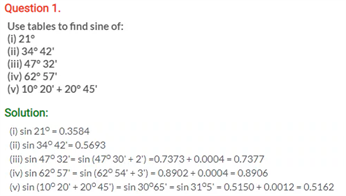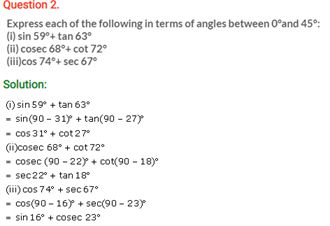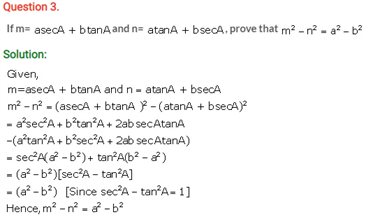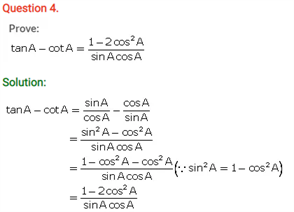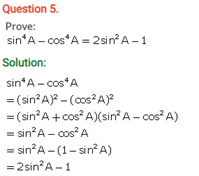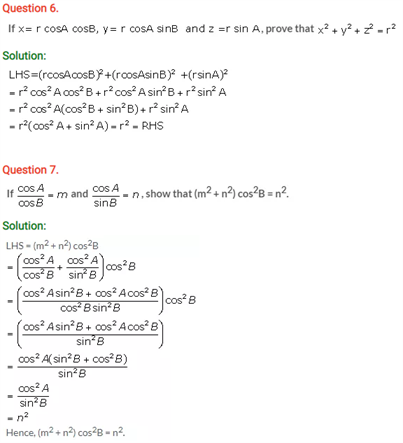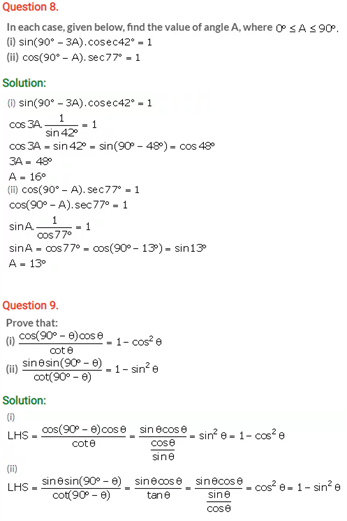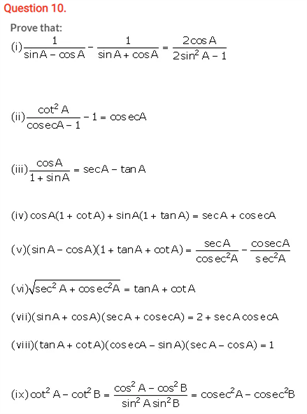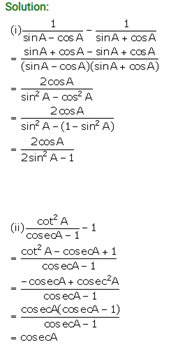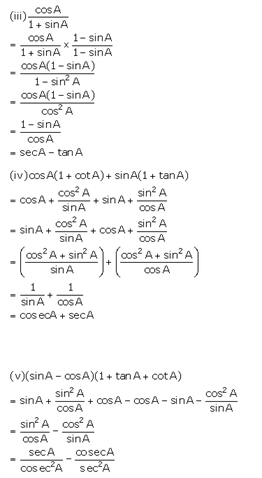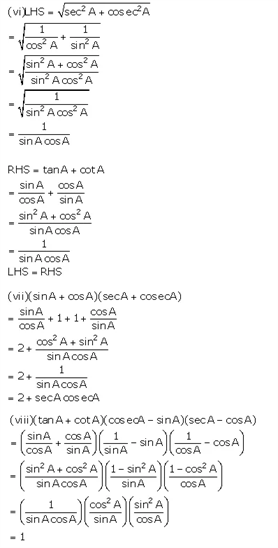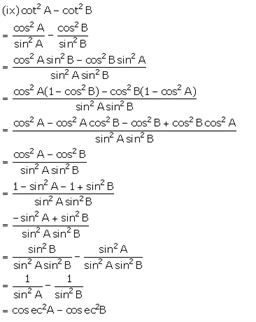# Selina Concise Maths Solution for ICSE Class 10 Chapter 21 Trigonometrical Identities

## Selina Class 10 Maths Solutions for Chapter 21

ICSE Solutions For Class 10 Maths brings you the questions and answers from a very important branch of mathematics, trigonometry. Trigonometry primarily deals with triangles, mostly right triangles. In particular the ratios and relationships between the triangle’s sides and angles. It has two main ways of being used: In its geometry application, it is mainly used to solve triangles, usually right triangles. That is, given some angles and side lengths, we can find some or all the others. In a more advanced use, the trigonometric ratios such as Sine and Tangent, are used as functions in equations and are manipulated using algebra

Check out ICSE Class 10 Maths Selina Solutions Chapter 21 Trigonometric Identities below: